# MEL - a quick introduction

What follows is a five-part introduction to MEL, the 'Maya Embedded Language'. MEL is *not* object-oriented, it is pretty simple to learn.

Knowing MEL, maya.cmds *and* PyMEL will make you quite valuable in the world of CG production :)

Here is how the topics are organized into small subtopics:

• 1. Comments, variables, types (including arrays), operators
• 2. Expressions, flow-control (branching, looping, recursion)
• 3. 'procs' (wrappers for code)
• 4. Sample scripts, part I: modeling, animation, rendering
• 5. Sample scripts, part II: dynamics, UI; also, eval(), file-handling, expressions

# 1: Basics, variables, operators

## Basics

MEL characteristics:

• free-form
• whitespace - spaces, tabs, blank lines
• scripts are made up of 'procedures' which are made up of 'statements'
• semi-colons terminate statements
• comments are in two flavors: // and /* .. */
```/*
This is a comment  that spans multiple lines. Eg. you can include a para about how an algorithm (that follows these  comment lines) works. You can also use this style of commenting to create a block of 'attribution' data - wrote the  code, when, where, what the code does etc.
*/

// this is a one liner```

Throughout these pages of notes, what's shown against a light background is MEL code which you can copy and paste verbatim into your script ed. window, eg:

```sphere; // create a NURBS sphere..
```

## Variables

Variables form the “currency” of modern programming:

• placeholders for data [memory locations]
• just two operations - “get”, "put"
• name, type and a value
• \$..
• proper naming is essential to maintainability

Here is a way to picture a variable: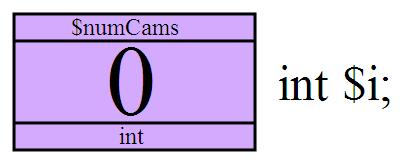Exactly five built-in variable types in MEL:

• int
• float
• string
• vector
• matrix

Of the above five types, only int, float, string and vector types can be arrays (see below). So MEL has a total of 9 built-in variable types: 5 'primitive' ones, 4 array ones. NO MORE! In other words, new var. types CANNOT be added (no 'structs' or 'classes').

## integer variables

```int \$i; int \$j=5;

int \$numCams = 24;

```

When we assign a (new) value to a var, that value is what is stored: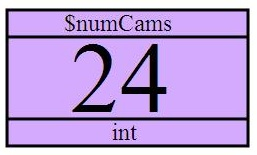```int \$k, \$l;
int \$i = 4.765; // will be truncated (NOT rounded) to 4
```

## float variables

```float \$f1;
float \$f1a, \$f1b;
float \$f2 = 6.65;
float \$f3 = 1/3; // \$f3 will be 0, NOT 0.3333333
float \$f4 = 1.0/3; // will be 0.333333333 as expected
```

## string variables

```string \$a;
string \$fnm = "Los ";
string \$lnm ="Angeles";
// concatenation; also note the use of a space character
string \$fullName = \$fnm + " " + \$lnm;
```

## vector variables

```vector \$v1 = <<1.0,0.56,-0.96>>;
print(\$v1.x); // NEED the ()
\$v1 = <<\$v1.x,\$v1.y,10.0>>; // NEED to reset all values, can't just set \$v1.z [see below]
\$v1.z = 10.0; // NOT allowed, syntax error
```

## matrix variables

```matrix \$n;
matrix \$i2 = <<1,2,3;-6,.78,.45>>; // 2 rows,3 columns. Note the use of ';' to separate rows
\$j2 = \$i2; // \$j2 is another matrix created with same contents as \$i2 [copied over]
\$k2 = -\$i2; // \$k2 is a new matrix with values from \$i2 NEGATED and copied over
```

• can +,-,*,/and % a matrix by a scalar [float or int]
• rows are separated by semicolons, columns by commas
• multiplication of matrices yields a new result matrix
• can negate a matrix
• indices start at 0, not 1
• to access an individual element, say '\$f = \$m`,etc.
• are not at all widely used :)

Deformations such as twist, taper and bend can be expressed in the form of matrices. Every vertex/CV of a shape can then be multiplied with such matrices to yield deformed shapes.

## Arrays

• ordered collections of primitive types [except matrices]
• used for lists, grouping data..
• can grow as needed [dynamic memory allocation]
• size(\$arrayname)
• clear() to delete
• access: [] to specify an index, eg. '\$nextNum = \$hugeArray;'
• indices start at 0, not 1
```int \$a2[];
int \$b2;
// here's how to initialize an array [specify all values while creating the variable]
float \$flArr= {0.8,-.6,1.,14.,12.3,-7.6};

clear \$b2; // empty out contents, size goes to 0
\$b2 = 1969; // this assignment will grow the size to 10000
```

## Operators

• symbols ["punctuation", informally speaking] that work with keywords and variables to form expressions
• for example, the assignment operator is '='

Operators come in three categories/'flavors' - arithmetic, comparison, logical [this is in addition to '=' (assignment) and ',' (comma) which are also operators].

### Arithmetic operators

```+
-
*
/
%

+=
-=
*=
/=
++ [equivalent to +=1]
-- [equivalent to -=1]
Note: there's no [need for a] '**' or '//' operator!
```

Variables to the left of an '=' sign RECEIVE values, ie are written into. Vars to the right behave as "read only", ie their values are accessed and used in calculations, but are not modified/written into.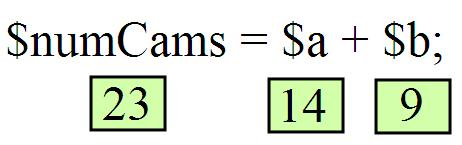### Relational [comparison] operators

These express relationships between two variables' contents (ie. values they contain).

```==
!=
>
<
>=
<=
```
```// The result of a comparison is captured in an int variable. Eg.
int \$areTheyIdentical = (\$a==\$b); // are 'a' and 'b' identical?
```

### Logical operators

The three 'logical' operators AND (&&), OR (||) and NOT (!) implement "Boolean" logic. These are HEAVILY used in loops and branches to express program logic, so you do need to understand these very, very well! Without logical operators, modern computer programming simply cannot exist.

In the following "truth tables", A and B are 'tests' (questions) where their answer is always a yes (1) or no (0). So are the results of using A and B along with the logical operators..

### && (AND, or 'intersection')

```A   B    A_AND_B

0   0    0
1   0    0
0   1    0
1   1    1
"A AND B" is true *ONLY IF* A is true and also B is true.
```

### || (OR, or 'union')

```A   B    A_OR_B

0   0    0
1   0    1
0   1    1
1   1    1
"A OR B" is true *IF EITHER* A is true or B is true (or both are true).
```

### ! (NOT, or 'inversion')

```A    NOT_A
0    1
1    0
'NOT A' is the opposite of A (duh!!).
```

Interestingly, as per "de Morgan's laws", we can use the pair (AND, NOT) to replace OR [likewise, the pair (OR, NOT) to replace AND]:

```'A OR B' identity (OR is expressed purely in terms of AND, NOT):
NOT(NOT(A) AND NOT(B)) = NOT(NOT(A)) OR NOT(NOT(B)) = A OR B

'A AND B' identity (AND is expressed purely in terms of OR, NOT):
NOT(NOT(A) OR NOT(B)) = NOT(NOT(A)) AND NOT(NOT(B)) = A AND B
```

In the above, A and B are tests, eg. A can be (\$a==\$c) and B can be (\$a>\$d). Using these as examples, the 'A AND B' identity above means this:

```int \$b;
\$b = (\$a==\$c) && (\$a>\$d); // A AND B
\$b = !( !(\$a==\$c) || !(\$a>\$d) ); // NOT( NOT(A) || NOT(B) )
```

If you create variables \$a, \$b, \$c, \$d, provide values for \$a, \$c and \$d, then run the above two MEL expressions, you'll find that \$b comes out to be the same in both cases (both will come out 1 or come out 0). Doing verifies the 'A AND B' identity for us.

So if the && operator is banished from MEL (or from all programming languages in the world!) we can make do with ||. Likewise, if || goes away, we can use && instead. But ! CANNOT go away, it is ESSENTIAL. In other words "you cannot not use NOT!".

Exercise 1: use truth tables to prove the above two identities.

Exercise 2: verify the 'A OR B' identity above, using MEL, just like we did for 'A AND B'.

## Connections to set theory and logic gates

The &&, II and ! operators are related to logical circuits (the fundamental building blocks of computation!) and to set theory.

Here are the three logic "gates" [regular and IEC (International Electrotechnical Commission) symbols, truth table]: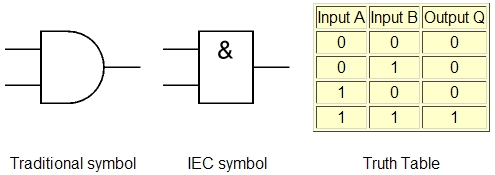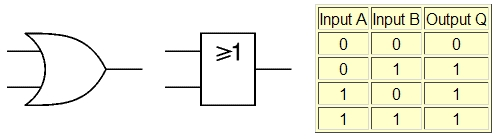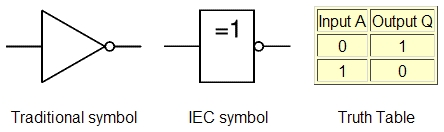Likewise, these are the set operations:For sets A and B (circles), their 'intersection' (shown in red) is equivalent to && - elements in the red area are in A *and* in B.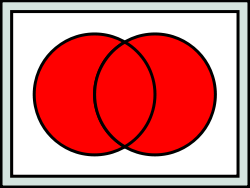The || operator is equivalent to set 'union' - an element can be in A *or* in B to be in the union set.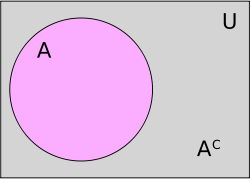The ! operator is like a set's complement (inversion) - it denotes elements (in gray) *not* in the set.

# 2: Expressions, flow control

## Expressions

Expressions make use of operators and variables, to form parts of statements..

In other words, a whole programming "sentence" is a 'statement'; a "phrase", an 'expression'. To put it differently, statements are comprised of expressions.

Here's an assortment of statements - pick out the expression(s) in each:

```// overall evaluation happens right to left
int \$i = \$j + \$k;
float \$f = 2.718;
int \$ang = 375 % 360; // modulus
int \$k = (\$j == \$r); // always 0 or 1
int \$v = ( (\$f == \$a) && (\$g < \$s) );
\$i = 45; // OK
45 = \$i; // *not* OK!
\$i = (\$f==3.1415); // OK
\$i = (3.1415==\$f); // also OK
```

## Flow control - overall idea

Flow constructs:

• help express program logic
• can be deeply nested (telescoped) inside each other
• make “real” programming happen [without them, all we'll have are sequential chains of statements].

Flow constructs help BREAK UP THE LINEARITY of statements/logic!

## Flow control - two (or three, including "recursion") ways in MEL

The three flow-related constructs are:

• looping
• branching
• recursion

Due to flow control constructs, we need a 2D surface (not just a line) to express program logic using diagrams.

### Looping

Two (three) ways:

• 'for' statement: two versions
• 'while' statement

### Branching

Three variations:

• 'if-else' - binary branching
• 'case' - multiway branches
• conditional assignment, using the '?' operator (and the ':' operator)
```// if (\$a==6)\$k=5;else \$k=556;
int \$k = (\$a==6)?5:556;
```

#### Fun

"Should I stay or should I go?"

Courtesy of Eric Keller:

```if (\$go)
{
\$trouble = 1;
}
else
{
\$trouble = \$trouble*2;
}
```

### Recursion

Another powerful, elegant way to manipulate program flow is recursion, which MEL supports. Here's an example:

```// first, a non-recursive version of factorial:
proc int iterativeFactorial(int \$n)
{
int \$result = 1, \$i;

for(\$i=1;\$i<=\$n;\$i++)
{
\$result *= \$i;
}

return \$result;
}// iterativeFactorial(()

// run the non-recursive (loop-based) version:
int \$rslt = iterativeFactorial(6);

// next, a 'recursive' version - it's called that because we use
// recursiveFactorial() // in its own definition, ie. we are
// explaining/defining something by "using itself" to do so
proc int recursiveFactorial(int \$n)
{
if(\$n==0)
return 1; // 0!=1, by definition
else
return (\$n*recursiveFactorial(\$n-1)); // recursion!!
}// recursiveFactorial(()

// run the recursive version
int \$rslt = recursiveFactorial(6);
```

Recursion is very good for creating certain kinds of snowflake curves, tiling patterns, cloud/fireball shapes, plants/trees, etc.

## Flow control - summary

To recap, ‘flow’ refers to flow of program statements, which really reflect flow of logic.

Before you write a single line of MEL, make sure that you can write your program out in pseudocode on index cards, run through the main parts in your head and explain it to your non-technical neighbor.

DESIGN, **then** CODE. Coding is the “easy” part..

## Extras

```// "horn"
int \$theta;
for(\$theta=0;\$theta<360;\$theta+=10)
{
float \$r=1.0;
float \$x = \$r*cos(\$theta*3.14159278/180);
float \$y = \$r*sin(\$theta*3.14159278/180);
sphere -p \$x \$y 0.0 -r (.05+(\$theta*.001));
}
```
```// grid of spheres
for(\$i=0;\$i<5;\$i++)
{
for(\$j=0;\$j<5;\$j++)
{
sphere -r .1 -p \$i \$j 0.0;
}
}
```
```// box of spheres
proc boxOfSpheres(int \$nS, float \$rMin, float \$rMax,int \$size)
{
int \$i;
for(\$i=0;\$i<\$nS;\$i++)
{
float \$x = rand(-\$size,\$size);
float \$y = rand(-\$size,\$size);
float \$z = rand(-\$size,\$size);
float \$r = rand(\$rMin,\$rMax);
print("rad is " + \$r + "\n");
sphere -p \$x \$y \$z -r \$r;
}
}// boxOfSpheres

// usage:
boxOfSpheres(100,1,2,10);
```
```// spiral phyllotaxis
float \$goldenAngle = 137.5*3.14159278/180.0;
int \$n=500;

for(\$i=1;\$i<=\$n;\$i++) {
float \$r = \$c*sqrt(\$i);
float \$a = \$goldenAngle*\$i;

float \$x=\$r*cos(\$a), \$y=\$r*sin(\$a);

cylinder -ax 0 0 1 -hr 2 -d 3   -r \$seedRad; // -s 8 -nsp 1
move \$x \$y 0;
}// next \$i
```

# 3: MEL procs

## Procedures, or "procs"

From now on, start thinking about programming problems in terms of inputs and outputs, NOT in terms of variables, for() loops, etc. In other words, think in terms of your problem, not in terms of programming language constructs.

Putting it another way, programming is not as easy as ABCD, but it *is* as easy as BDCA:

• Brainstorm
• Design
• Code

Specifically, design before you start coding. This advice holds for any language/OS, any software development project. The idea is to not get caught up in details right at the start. "Big picture first", "top down programming", "divide and conquer", "don't lose the forest for the trees" - all these phrases are other ways of saying the same thing.

In MEL you can create well-structured programs by using a construct called a 'proc'. Procedure, function, subroutine, method - these are names used to denote the same concept in other programming languages.

Notes on MEL procs:

• a proc is a self-contained body of code, a 'wrapper' around 'raw' MEL; it also serves to give the raw code a name (which can be used later to access the code)
• it is a packaging mechanism, to allow for connect-the-boxes/input-output/dataflow/LEGO-style programming; a user of the proc (yourself or someone else) simply knows HOW to use it, not WHAT is inside - in other words, it's a "black box"
• use of procs permits creation of clean, modular, easily readable/extensible code
• also permits code reuse and bug isolation
• procs can 'talk' to each other (a proc can make use of other procs) and with Maya and with the user - THIS IS THE HEART OF PROGRAMMING!!!

A proc is like a new 'word' that needs to be 'taught' to Maya, the same way a child is taught the meaning of a new word. So a proc needs to be created/defined/declared to Maya before it can be used/called/executed. The declaration happens just once, and from then on, the calling can happen any number of times. It is an error to use a proc before defining it, just like it doesn't make sense in a long novel to start talking about a character at length before the character has been introduced to the reader.

MEL programming can be summarized as 'writing procs that communicate with each other via their inputs and outputs'. In the process, useful things happen to/in your scene (things get created, deleted, modified).

## Structure (syntax) of a proc

```// proc syntax
// things in square brackets (INCLUDING the square brackets!) are OPTIONAL,
// ie. you can leave them out if necessary
[global] proc [return_type] proc_name ([args])
{
[MEL stmts]
}
```

## Inputs, outputs

```
// the SIMPLEST MEL proc possible - NO inputs, no output, no code inside
proc doNothing()
{

}

// a proc that is a 'wrapper' for the built-in 'sphere' command
proc mkSph()
{
sphere; // NOT an output but a 'side effect'
}
```
```
proc printHi()
{
print("Hello MEL world!\n"); // NOT an output, another side effect
}
```
```// With inputs, but no output
{
int \$sum = \$a+\$b;
print("Sum: " + \$sum + "\n"); // again, NOT an output
}

```
```
// Full-blown case: inputs AND output
proc int addAandB(int \$a, int \$b)
{
int \$sum = \$a+\$b;
print("Sum: " + \$sum + "\n"); // NOT an output
return \$sum; // THIS is what constitutes output
}
```
```
// again, has input AND output
proc string printAge(int \$howOld)
{
string \$rslt;
\$rslt = "You are " + \$howOld + " years old\n";
return \$rslt; // note - we *could* combine all three stmts here into just one [how?!]
}// printAge()
```

A proc can have any NUMBER and TYPE (from the 9 MEL types) of inputs, but ONLY ONE output (again from the 9 MEL types). The aim of the proc is to compute that output to return. What to do with output (return value)? CAPTURE IT, ie. store it in our own variable. That variable can now input to ANOTHER proc, etc. Output of one proc can become an input, even for multiple procs!

```
// output is lost (we're not receiving and storing it in a variable)
printAge(40);

// compared to above, output is captured (stored) into our own var called \$myAge
string \$myAge = printAge(36);
// ALTERNATE syntax to capture a proc's result:
string \$myAge = `printAge 36`;
```

You can either use the () notation or the ` ` notation to capture the result (output) of a proc, they are totally equivalent in terms of what happens.

```
// define another proc, to "wrap" print() into a useful debugging proc
proc prtStr(string \$s)
{
print("Debug: " + \$s + "\n");
}

// output from `printAge 36` captured in
// string \$myAge = `printAge 36`
// becomes new input to prtStr.
string \$myAge = `printAge 36`; // output from `printAge 36`is captured and stored in \$myAge
prtStr(\$myAge); // \$myAge becomes an input to prtStr (which itself has no output)

// alternative to above , without using the \$myAge variable:
prtStr(`printAge 36`); // or equivalently, prtStr(printAge(36));
```

Summary: Chaining (making one proc's output to be another's input) is what it's *ALL* about! Write useful, reusable units of code (procs) with good inputs and outputs, then hook them up :) This is how programming gets done in every prog. language.

## Code refactoring (for efficiency/better organization)

You should always strive to make your procs more lucid/efficient/compact etc., while keeping the input/output "signature" (input TYPES in their proper sequence, and output TYPE) unaltered. Doing so is called "code refactoring".

One example is a program to add first N natural numbers, ie. 1+2+3+...N. Two ways to do this:

• using a for() loop
• using this formula instead: N*(N+1)*0.5
```
// Try the following example using the brute force method as
// well as Gauss' formula (both given below), and compare running times:
// sumOfFirstNNaturalNumbers 1007785;

// Brute force method
global proc int sumOfFirstNNaturalNumbers(int \$n)
{
int \$sum = 0, \$i;

float \$startTime = `timerX`;

for(\$i=1;\$i<=\$n;\$i++)
\$sum += \$i;
// timeElapsed will receive the diff. between current time and
// the stored 'startTime' value..
float \$timeElapsed = `timerX -startTime \$startTime`;
print ("Time taken: "+\$timeElapsed + "\n");

return \$sum;
}

// Gauss' 'constant time' method
global proc int sumOfFirstNNaturalNumbers(int \$n)
{
float \$startTime = `timerX`;
int \$sum = (0.5*(\$n)*(\$n+1)); // NO NEED FOR A LOOP!!
float \$timeElapsed = `timerX -startTime \$startTime`;
print ("Time taken: "+\$timeElapsed + "\n");

return \$sum;

}
```

In the above, the for() loop version takes longer and longer as the input number gets bigger. In contrast, Gauss' clever formula takes the SAME, FIXED amount of time, regardless of input size!

Another example is an 'add4Nums()' proc which will add four floats that are input to it. Assuming that an 'add2Nums' proc is known to MEL, we can successively refine our approach like so:

```
// Make sure we have this first:
proc float add2Nums(float \$a, float \$b)
{
return (\$a+\$b);

// Now we can start writing add4Nums()

// version 0
proc float add4Nums(float \$a, float \$b, float \$c, float \$d)
{
float \$sum = \$a + \$b + \$c + \$d;
return \$sum;
}
// Eg.:
// add4Nums 0.1 0.2 -.2 -.1;

// version 0.5
proc float add4Nums(float \$a, float \$b, float \$c, float \$d)
{
return (\$a + \$b + \$c + \$d); // no need for a \$sum variable
}

// version 1
proc float add4Nums(float \$a, float \$b, float \$c, float \$d)
{
float \$sum1 = add2Nums(\$a,\$b); // CALL another proc!
float \$sum2 = add2Nums(\$c,\$d); // CALL another proc!
return (\$sum1+\$sum2);
}

// version 2
proc float add4Nums(float \$a, float \$b, float \$c, float \$d)
{
float \$sum1 = add2Nums(\$a,\$b); // CALL another proc!
float \$sum2 = add2Nums(\$c,\$d); // CALL another proc!
return add2Nums(\$sum1,\$sum2); // no '+' sign anywhere in this proc :)

// version 3 - the MOST COMPACT way to do this!
proc float add4Nums(float \$a, float \$b, float \$c, float \$d)
{
}
```

## Storing code in MEL scripts (vs. typing it in each time via the Script Editor)

MEL scripts are simply text files that contain MEL code (usually inside procs). We place code in files on disk, in order to avoid typing it all in each time we restart Maya.

In order for Maya to automagically run your proc from a file ("MEL script"), follow these steps:

• create a **global** proc [the 'global' keyword is what lets Maya load your proc from a file].
• name the MEL script (file) to be the same name as your proc.
• place the MEL script in a 'known' place (one of the directories in the list output by `getenv MAYA_SCRIPT_PATH`). If you want you can use the 'putenv' command to add any arbitrary directory (containing MEL scripts you want Maya to locate) to this list.
• bring up a fresh instance of Maya and simply call the proc, no need to declare it in the Script Editor! THIS IS MAGIC. It means scripts containing useful procs can 'live' anywhere in the filesystem, and Maya can automagically locate them, load them and run them for you.
• if you now make changes to a MEL script and want [already-running] Maya to run your updated procs(s), you need to 'source' the program in order to make Maya pick up your change, then re-run your proc. So the software dev. cycle is 'edit->save->source->run, edit->save->source->run, edit->save->source->run....'. You can package the source,run commands into a shelf button and click on it each time you modify your script and save it.
• if you create a brand new MEL script *after* having brought up Maya, you need to run 'rehash;' to inform Maya about your program, and *then* run the proc(s) in the script as mentioned above.

To run a MEL proc. from an arbitrary location (eg. Desktop, C:/temp etc. on a PC), use the 'source' directive (the same command mentioned above, to refresh a proc in Maya after you've changed it on disk).

Now that you know about procs calling other procs, the 'timer' code from the 'sumOfFirstNNaturalNumbers' proc above (either version) can be abstracted into a separate proc (which could also be used elsewhere).

```// Brute force method
global proc int sumOfFirstNNaturalNumbers(int \$n)
{
int \$sum = 0, \$i;

float \$startTime = `timerX`;

for(\$i=1;\$i<=\$n;\$i++)
\$sum += \$i;
// timeElapsed will receive the diff. between current time and
// the stored 'startTime' value..
float \$timeElapsed = `timerX -startTime \$startTime`;
print ("Time taken: "+\$timeElapsed + "\n");

return \$sum;
}
```

We can split the above up (refactor it), like so:

```// prints how much time has elapsed, given a start time via the \$startTime incoming variable
proc printElapsedTime(float \$startTime)
{
float \$timeElapsed = `timerX -startTime \$startTime`;
print ("Time elapsed: "+\$timeElapsed + "\n");
}

// in our main adding proc we'll simply "call" the printElapsedTime proc
global proc int sumOfFirstNNaturalNumbers(int \$n)
{
int \$sum = 0, \$i;

float \$startTime = `timerX`; // start the stopwatch timer

for(\$i=1;\$i<=\$n;\$i++)
\$sum += \$i;

// all done with the calculation, how long did it take?
printElapsedTime(\$startTime);

return \$sum;
}
```

You can see that the above is 'cleaner' since we've separated the reporting (of time taken) from the adding. To time any piece of your own code, do:

```float \$startTime = `timerX`; // stopwatch START

// ..

printElapsedTime(\$startTime); // stopwatch STOP and PRINT
```

In a production environment, such a piece of code (the 'printElapsedTime' proc) might be placed in its own file (printElapsedTime.mel) which would then be kept in a 'known' directory [note that the printElapsedTime proc needs to be global!]. That done, any other MEL script can simply use the code just by calling it, as outlined above. Over time a large collection of useful procs can be built up, creating a productive 'ecosystem'. Studios such as DreamWorks, Disney, ILM, Sony etc. tend to have thousands of such MEL scripts in their repositories :)

## MEL commands

Commands are like procs that come with (are built into) Maya.

• over a thousand of these commands exist, new ones are added for each new Maya version (look in the Help docs for the complete list)
• treat them as built-in procs
• commands provide 'hooks' into the graphical aspects of Maya, something our own MEL simply cannot provide [in other words, it is not possible just using MEL to write a proc that will draw a sphere on the screen, whereas the built-in 'sphere' command does just that]
• HUGE diff. compared to user-created procs: commands can have a VARIABLE number of inputs!
• commands can be called (used) inside your own proc definitions. This is what makes MEL a 3D CG language compared to Perl, JavaScript, etc. In other words, commands AMPLIFY the power of MEL. Or turning this around, MEL exists as a programming language wrapper for these commands.

## A pair of add'l commands

'eval'/'evalEcho' - to execute contents of a string var. EXTREMELY USEFUL for procedurally (ie using code, specifically, loops and branches) creating curves, particle systems, geometry, etc.

```eval("cone;");
eval("sphere -r 2;";
eval("curve -d 1 -p 0 0 0 -p 1 0 0 -p 1 1  0 -p 0 1 0;");
```

'system' - to invoke arbitrary system commands.

```system "firefox";

// VERY bad/dangerous - if Perl is installed, running this on a PC
// *will* format the C drive *without* prompting for Y/N!!!!!
system "perl \"echo y ¦ format C:\""
```

Particularly on a Unix machine, system() is very useful for sending mail from within a MEL script, displaying a browser help page, doing some printing, etc.

Here's a situation where eval() is indispensable:

```// Eg. hairBall(100);
// Eg. crumpledPaper 20;

proc crumpledPaper(int \$n)
{
//file -f -new;
int \$i;
string \$hBalls[];
for(\$i=0;\$i<\$n;\$i++)
{
string \$hairB = hairBall(100);
float \$x = rand(-1,1);
float \$y = rand(-1,1);
float \$z = rand(-1,1);

move \$x \$y \$z;
print(\$hairB + "\n");
\$hBalls[size(\$hBalls)] = \$hairB; //!!!
}
select \$hBalls;
loft;
}

proc string hairBall(int \$numPts)
{
string \$curvCmd = "curve -d 3 ";
int \$i;
for(\$i=0;\$i<\$numPts;\$i++)
{
float \$x = rand(-1,1);
float \$y = rand(-1,1);
float \$z = rand(-1,1);

\$curvCmd += " -p "+\$x+" "+\$y+" "+\$z;
}
// string \$hB = evalEcho(\$curvCmd);
string \$hB = `evalEcho \$curvCmd`;
return \$hB;
}
```

## Extras

Here is a program to calculate 'Fibonacci numbers' and print ratios between consecutive Fibonacci numbers.

```// Eg. Fibonacci(30);
proc Fibonacci(int \$n)
{
int \$fSeq[] = {0,1}, \$i;
float \$gRatio[];

for(\$i=2;\$i<=\$n;\$i++)
{
\$fSeq[\$i] = \$fSeq[\$i-1] + \$fSeq[\$i-2];
\$gRatio[\$i] = float(\$fSeq[\$i])/\$fSeq[\$i-1];
}

int \$j;
for(\$j in \$fSeq)
{
print(\$j + "\n");
}
float \$k;
for(\$k in \$gRatio)
{
print(\$k + "\n");
}
}
```

# 4: Sample MEL scripts, part 1

## Deconstructing existing programs

What follows are a couple of programs each from the areas of modeling, animation and rendering. Play with each one for a bit, then analyze it for the following:

• use of vars, expressions
• flow control - branches, loops
• use of procs and built-in MEL commands, and how they are chained via input/output
• math/CG algorithms where applicable (not all MEL programs need to have these)
• programming 'style' (syntax and semantics)
• overall programming "logic" - how does everything work together to get the job done?
• ways to improve the program - more features? less features? better organization? better use of memory? better algorithms? ANY program can be made better in SOME way!!
• comments - useful? superfluous? verbose? terse?

By analyzing and adapting ("embrace, extend") existing programs you can start to develop your own bag of tricks, your own style and eventually, your own mastery of MEL.

## Nodes, connections

Underneath it all, a Maya scene is a collection of 'nodes' (that store data), hooked together via 'connections' using the nodes' 'attributes'.

So a lot of MEL programming understandably has to do creating/deleting nodes, creating/deleting node attributes, getting/setting attribute values, connecting/disconnecting attribute connections.

In addition to dealing with nodes, MEL programming also has to do with the following (which ultimately still involve nodes and their attributes):

• grouping, ungrouping, parenting, unparenting
• selecting
• querying/listing scene data
• dealing with the history mechanism (undo/redo)
• file I/O, rendering..
• setting up expressions, scriptJobs..
• dealing with user prefs
• UI creation
• other

## Rendering

Here is a way to rename materials:

```string \$newName = "New_Name";

string \$removeMat[] = {"lambert1", "particleCloud1", "initialShadingGroup", "initialParticleSE"};

string \$materials[] = stringArrayRemove(\$removeMat,`ls -mat`);

for(\$material in \$materials)
{
rename \$material \$newName;
print ("Renaming " + \$material + "\n");
}
```

Create a scene with some surfaces and a dir. light, choose 'lambert1' in your scene, then run:
colorizeUsingRamp;

abDropSpotCam - creates a light or camera at current viewcam's location.
abDropSpotCam <0|1|2|3|4>; // 0 or 1 or 2 or 3 or 4

paintRandom
Create a paintable surface, select it, choose a brush, then run:
paintRandom 10 10 1 0 10 0 1 0;# 5: Sample MEL scripts, part 2

## UI

Interact with Maya from a web browser - obsolete but COOL!!!!

## Expressions

These are just 'regular' MEL (with vars, flow control, procs..) typed inside the expression editor window, with pre-defined vars such as time and frame. An expression can also be created using the 'expression' (or 'dynExpression') MEL commmand.

```
Ball.translateX = Cube.translateX + 5;
Cube.translateZ = time;
Ball.scaleX = 1 + time;
Cone.scaleY = (0.5 + sin(time)*5);
if (time<3.0)
{
Ball.scaleY = time;
}
```

What does the following expression (expressed as a MEL statement) do?

```expression -e -s "catch(delete(\"nurbs*\"));\nsphere -r 0.2;\nfloat \$x = rand(4), \$y=rand(4), \$z=rand(4);\nmove \$x \$y \$z;"  -o "" -ae 1 -uc all  expression1;

```

Here is another example. As you can see, 'xform' (and other cmds) will also work inside an expression :)

```file -f -new;

sphere;

sphere;
move 1 2 3;

playbackOptions -e -min 0;
playbackOptions -e -max 100;

expression -s "string \$cv = \"nurbsSphere1.cv[\" + frame + \"]\";\n\nfloat \$cvPosition[] = `xform -q -ws -t \$cv`;\n\n\n\$cv = \"nurbsSphere2.cv[\" + frame + \"]\";\n\nxform -ws -t  \$cvPosition \$cvPosition \$cvPosition  \$cv;"  -o nurbsSphere2 -ae 1 -uc all ;

play -st 1;
```